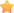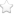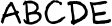×1. A+E < C+5
2. A+C < E
3. A+C+E=D
4. A+D=B
5. D>6
6. C是D的因數• A=1
• B=9
• C=2
• D=8
• E=5

D=7 or 8

（你有更好的解法嗎？歡迎到粉絲團遊戲學校去跟大家分享喔！）